# The Kalman Filter. Helping Chickens Cross the Road.

David Austin
Grand Valley State University

I was running some errands recently when I spied the Chicken Robot ambling down the sidewalk, then veering into the bike lane. A local grocery chain needs to transport rotisserie chickens from one of its larger stores to its downtown market. Their solution? A semi-autonomous delivery vehicle that makes the three-mile journey navigating with GPS.

I was curious how this worked. The locations provided by most GPS systems are only accurate to within a meter or so. How could those juicy chickens know how to stay so carefully in the center of the bike lane?

A typical solution to problems like this is an elegant algorithm, known as Kalman filtering, that’s embedded in a wide range of technology that most of us frequently either use or benefit from in some way. The algorithm allows us to efficiently combine our expectations about the state of a system, the chickens’ physical location, for instance, with imperfect measurements about the system to develop a highly accurate picture of the system.

This column will describe some simple versions of Kalman filtering, the main observation that makes it work, and why it’s such a great idea.

### A first example

Let’s begin with a simple example. Suppose we would like to determine the weight of some object. Of course, any scale is imperfect so we might weigh it repeatedly on the same scale and find the following weights, perhaps in grams.

 50.4 46 46.1 45.1 48.9 42.6 50.7 45.7 47.8 46.7

As the result of each weighing is recorded, we update our estimate of the weight as the average of all the measurements we’ve seen so far. That is, after obtaining $z_n$, the $n^{th}$ weight, we could estimate the object’s weight by finding the average $$x_n = \frac1n(z_1 + z_2 + \ldots + z_n).$$ The result is shown below.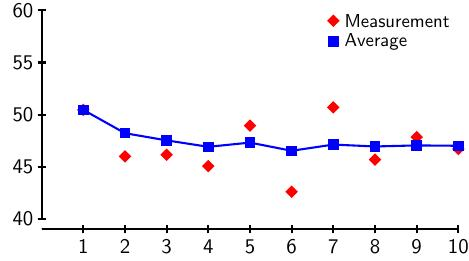As the next weight $z_{n}$ is recorded, we update our estimate:
\begin{aligned} x_{n} & = \frac1{n}\left(z_1 + z_2 + \ldots + z_{n}\right) \ x_{n} & = \frac1{n}\left((n-1)x_{n-1} + z_{n}\right) \ x_{n} & = x_{n-1} + \frac1{n}\left(z_{n} – x_{n-1}\right) \end{aligned}

This expression tells us how each new measurement influences the next estimate. More specifically, the new estimate $x_{n}$ is obtained from the previous estimate $x_{n-1}$ by adding in $\frac1{n}(z_{n} – x_{n-1})$. Notice that this term is proportional to the difference between the new measurement and the previous estimate with the proportionality constant being $K_n = \frac1{n}$. We call $K_n$ the Kalman gain; the fact that it decreases as we include more measurements reflects our increasing confidence in the estimates $x_n$ so that, as $n$ increases, new measurements have less effect on the updated estimates.

Suppose now that we have some additional information about the accuracy of our measurements $z_n$. For example, if $z_n$ is the chickens’ location reported by a GPS system, the true location is most likely within a meter or so. More generally, we might imagine that the true value is normally distributed about $z_n$ with standard deviation $\sigma_{z_n}$.

Returning to our weighings, we could perhaps estimate $\sigma_{z_n}$ by repeatedly weighing an object of known weight. The probability that the true value is near $z_n$ is given by the distribution
$$p_{z_n}(x) = \frac{1}{\sqrt{2\pi\sigma_{x_n}^2}} e^{-(z-z_n)^2/(2\sigma_{z_n}^2)}.$$

Let’s also suppose that we have some estimate of the uncertainty of our estimates $x_n$. In particular, we’ll assume the probability that the true value is near $x_n$ is given by the normal distribution having mean $x_n$ and standard deviation $\sigma_{x_n}$:
$$p_{x_n}(x) = \frac{1}{\sqrt{2\pi\sigma_{x_n}^2}} e^{-(x-x_n)^2/(2\sigma_{x_n}^2)}.$$
We’ll describe how we can estimate $\sigma_{x_n}$ a bit later.

These distributions could be as shown below.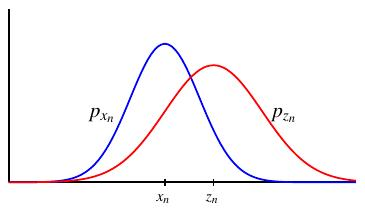Now here’s the beautiful idea that underlies Kalman’s filtering algorithm. Both distributions $p_{x_n}$ and $p_{z_n}$ describe the location of the true value we seek. We can combine them to obtain a better estimate of the true value by multiplying them. That is, we combine or “fuse” these two distributions using the product
$$p_{x_n}(x) p_{z_n}(x).$$
Since the product of two Gaussians is a new Gaussian, we obtain, after normalizing, a new normal distribution that better reflects the true value. In our example, the product $p_{x_n}p_{z_n}$ is shown in green.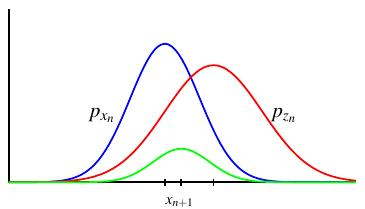More specifically, the product of the two distributions is
\begin{aligned} & \exp\left[-(x-x_n)^2/(2\sigma_{x_n}^2)\right] \exp\left[-(x-z_n)^2/(2\sigma_{z_n}^2)\right] \ & \hspace{48pt}= \exp\left[-(x-x_n)^2/(2\sigma_{x_n})^2-(x-z_n)^2/ (2\sigma_{z_n}^2)\right]. \end{aligned}
Expanding the quadratics in the exponents and recombining leads to the new normal distribution
$$\frac{1}{\sqrt{2\pi\sigma_{x_{n+1}}^2}} \exp\left[-(x-x_{n+1})^2/(2\sigma_{x_{n+1}}^2)\right]$$
where
\begin{aligned} x_{n+1} & = \frac{\sigma_{z_n}^2}{\sigma_{x_n}^2+\sigma_{z_n}^2} x_n + \frac{\sigma_{x_n}^2}{\sigma_{x_n}^2+\sigma_{z_n}^2} z_n \ & = x_n + \frac{\sigma_{x_n}^2}{\sigma_{x_n}^2+\sigma_{z_n}^2}(z_n – x_n) \ \end{aligned}
Notice that this expression is similar to the one we found when we updated our estimates by simply taking the average of all the measurements we have seen up to this point. The Kalman gain is now
$$K_n = \frac{\sigma_{x_n}^2}{\sigma_{x_n}^2+\sigma_{z_n}^2}$$

In addition, the product of the Gaussians leads to the new standard deviation
$$\sigma_{x_{n+1}}^2 = \frac{\sigma_{x_n}^2\sigma_{z_n}^2}{\sigma_{x_n}^2+\sigma_{z_n}^2} = (1-K_n) \sigma_{x_n}^2$$

Notice that the Kalman gain satisfies $0\lt K_n \lt 1$. In the case that $\sigma_{x_n} \ll \sigma_{z_n}$, we feel much more confident in our estimate $x_n$ than our new measurement $z_n$. Therefore, $K_n\approx 0$ and so $x_{n+1} \approx x_n$, reflecting the fact that the new measurement has little influence on our next estimate.

However, if $\sigma_{x_n} \gg \sigma_{z_n}$, we feel much more confident in our new measurement $z_n$ than in our estimate $x_n$. Then $K_n\approx 1$ and $x_{n+1}\approx z_n$.

In both cases, the uncertainty in our new estimate, as measured by $\sigma_{x_{n+1}}^2 = (1-K_n)\sigma_{x_n}^2$, has decreased.

This leads to the following algorithm:

1. Initialize the first estimate $x_1$ and the uncertainty $\sigma_{x_1}$. We could use the first measurement as the first estimate or we could simply make a guess for the estimate and choose a large uncertainty.
2. Each time a new measurement $z_{n}$ arrives, update the estimate $x_{n+1}$ nd uncertainty $\sigma_{x_{n+1}}$ as follows:
• Find the Kalman gain $K_n = \frac{\sigma_{x_n}^2} {\sigma_{x_n}^2+\sigma_{z_n}^2}$.
• Update our estimate $x_{n+1} = x_n + K_n(z_{n}-x_n)$.
• Update our uncertainty $\sigma_{x_{n+1}}^2 = (1-K_n)\sigma_{x_n}^2$. Since $0\lt 1-K_n\lt 1$, the uncertainty will continually decrease, which makes the algorithm relatively insensitive to the initialization.

To illustrate, suppose that we make many measurements of a known weight with our scale and determine that the standard deviation of its measurements is constant $\sigma_z=2$. Initializing so that $x_1 = z_1$ and $\sigma_{x_1} = \sigma_z = 2$, we have the following sequence of estimates along with the decreasing
sequence of uncertainties.### Tracking a dynamic system

In our first example, the quantity we’re tracking, the weight of some object, doesn’t change. Let’s now consider a dynamic process where the quantities are changing. For instance, suppose we’re tracking the height and vertical velocity of a moving object. The true height of the object could look like this, although
that true height is not known to us.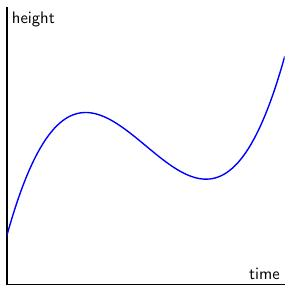In addition to recording the height $h$, we will also track the vertical velocity $v$ so that the state of the object at some time is given by the vector
$${\mathbf x}_{n,n} = \begin{bmatrix} h_n \ v_n \end{bmatrix}.$$
The reason for writing the subscript in this particular way will become clear momentarily.

Moreover, we will assume that there is some uncertainty in both the position and the velocity. Initially, these uncertainties may be uncorrelated with one another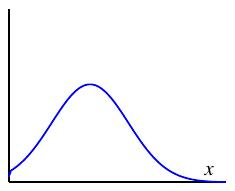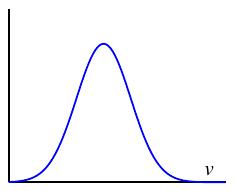so that we arrive at a Gaussian
$$\exp\left[-(h-h_n)^2/(2\sigma_{h_n}^2) – (v-v_n)^2/(2\sigma_{v_n}^2)\right]$$
that describes the multivariate normal distribution of the true state. A more convenient expression for this Gaussian uses the covariance matrix
$$P_{n,n} = \begin{bmatrix} \sigma_{h_n}^2 & 0 \ 0 & \sigma_{v_n}^2 \ \end{bmatrix}$$
so that the Gaussian is defined in terms of the quadratic form associated to $P_{n,n}^{-1}$:
$$\exp\left[-\frac12 ({\mathbf x} – {\mathbf x}{n,n})^TP{n,n}^{-1}({\mathbf x} – {\mathbf x}_{n,n})\right].$$
The following figure represents the value of this distribution by shading more likely regions more darkly.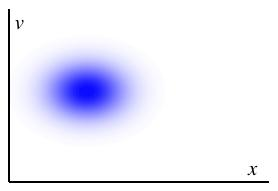Now if we know $x_{n,n}$, the state at some time, we can predict the state at a later time using some assumption about the system. For instance, we might assume, after $\Delta t$ time units have passed, that the new state is ${\mathbf x}{n+1,n} = \begin{bmatrix}h{n+1} \ v_{n+1} \end{bmatrix}$ where
\begin{aligned} h_{n+1} & = h_n + v_n\Delta t \ v_{n+1} & = v_n. \end{aligned}
That is, we assume that the height has increased at the constant velocity given by the state vector ${\mathbf x}n$ and that the velocity has remained constant. More succinctly, if
$$F_n = \begin{bmatrix} 1 & \Delta t \ 0 & 1 \end{bmatrix},$$
we can write
$${\mathbf x} {n+1, n} = F_n{\mathbf x}{n}.$$
The subscript ${\mathbf x} {n+1, n}$ reflects the fact that we are extrapolating the next state using information only from the previous state.

This is a particular model we are choosing; in other situations, another model may be more appropriate. For instance, if we have information about the object’s acceleration, we may want to incorporate it. In any case, we assume there is a matrix $F_n$ from which we extrapolate the next state:
$${\mathbf x}{n+1,n} = F_n{\mathbf x}{n,n}.$$

Of course, uncertainty in the state ${\mathbf x}{n,n}$ will translate into uncertainty in the extrapolated state ${\mathbf x}{n+1,n}$. It is straightforward to verify that the covariance matrix is transformed as
$$P_{n+1,n} = F_nP_{n,n}F_n^T.$$
For instance, if the uncertainties in position and velocity are initially uncorrelated, they may become correlated after the transformation, which is to be expected.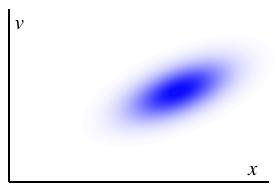$P_{n,n}$ $P_{n+1,n}$

These two transformations form the extrapolation phase of the algorithm:
\begin{aligned} {\mathbf x}{n+1,n} & = F_n{\mathbf x}{n,n} \ P_{n+1,n} & = F_nP_{n,n}F_n^T. \end{aligned}

Next, suppose we have a new measurement ${\mathbf z}{n}$ whose uncertainty is described by the covariance matrix $R{n}$. We imagine that the normal distribution centered on ${\mathbf z}_{n}$ and with covariance matrix $R_n$ describes the distribution of the true state.

As before, we will fuse our predicted state ${\mathbf x}{n+1,n}$ with the measured state ${\mathbf z}{n}$ by multiplying the two normal distributions and rewriting as a single Gaussian. With some work, one finds the expression for the Kalman gain $$K_{n} = P_{n+1,n}(P_{n+1,n} + R_{n})^{-1},$$ which should be compared to our earlier expression.

We also obtain the updated state and covariance matrix
\begin{aligned} {\mathbf x}{n+1, n+1} & = {\mathbf x}{n+1,n} + K_{n}({\mathbf z}{n} – {\mathbf x} {n+1,n}) \ P_{n+1,n+1} & = P_{n+1, n} – K_{n}P_{n+1,n}. \ \end{aligned}

So now we arrive at a new version of the algorithm:

1. Initialize the initial state ${\mathbf x}_{1,1}$ and covariance matrix $P_{1,1}$.
2. As new measurements become available, repeat the following steps:
• Form the extrapolated state and covariance:
\begin{aligned} {\mathbf x}_{n+1,n} & = F_n{\mathbf x}_{n,n} \\ P_{n+1,n} & = F_nP_{n,n}F_n^T. \end{aligned}
• Use the result to find the Kalman gain
$$K_{n} = P_{n+1,n}(P_{n+1,n} + R_{n+1})^{-1},$$
• Use the Kalman gain to fuse the predicted state with the measured state and update the covariance matrix.
\begin{aligned} {\mathbf x}_{n+1, n+1} & = {\mathbf x}_{n+1,n} + K_{n}({\mathbf z}_{n} – {\mathbf x}_{n+1,n}) \\ P_{n+1,n+1} & = P_{n+1, n} – K_{n}P_{n+1,n}. \\ \end{aligned}

Let’s see how this plays out in an example. Imagine we are tracking the height and vertical velocity of an object whose true height as is shown.Remember that we don’t know the true height, but we do have some noisy measurements that reflect the object’s height and its velocity.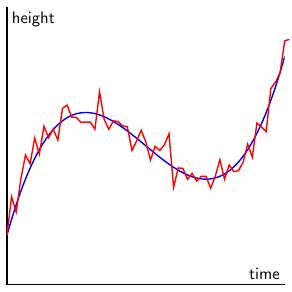Applying the Kalman filtering algorithm naively, we obtain the green curve describing the object’s height. Notice how there is a time lag between the filtered height and the true height.What’s the problem here? Clearly, the object is experiencing a significant amount of acceleration, which is not built into the model. As we saw in our earlier static example, the uncertainty in the estimated state ${\mathbf x}_{n,n}$ continually decreases, which means the confidence we have in our estimates grows and causes us to downplay the new measurements.

There are several ways to deal with this. If we have measurements about the acceleration, we could rebuild our model so that the state vector ${\mathbf x}{n,n}$ includes the acceleration in addition to the position and velocity. Alternatively, if we have information about how an operator is controlling the object we’re tracking, we could build it into the extrapolation phase using the update
$${\mathbf x} {n+1,n} = F_{n}{\mathbf x}_{n,n} + B_n{\mathbf u}_n$$
where ${\mathbf u}_n$ is a vector describing some additional control and $B_n$ is a matrix describing how this control feeds into the extrapolated state. Clearly, we need to know more about the system to incorporate a term like this.

Finally, we can simply build additional uncertainty into the model by adding it into the extrapolation phase. For instance, we could define
$$P_{n+1,n} = F_nP_{n,n}F_n^T + Q_n,$$
where $Q_n$ is a covariance matrix known as process noise and represents our way of saying there are additional influences not incorporated in the extrapolation model:
${\mathbf x}{n+1,n} = F_n{\mathbf x}{n,n}$.

Adding some process noise into the extrapolation phase prevents us from becoming overly confident in the extrapolated states and continuing to give sufficient weight to new measurements. This leads to the filtered state as shown below, which is clearly much better than the measured signal.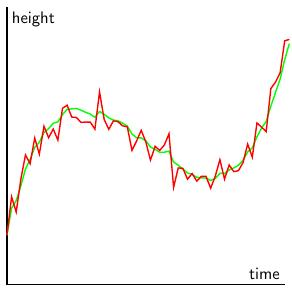### Summary

Kalman developed this algorithm in 1960, though it seems to have appeared earlier in other guises, and found a significant early use in the Apollo guidance computer. Indeed, the algorithm is well suited for this application. While the guidance computer was a marvel of both hardware and software engineering, its memory and processing power were modest by our current standards. As the algorithm only relies on our current estimate of the state, its demands on memory are slight, and the computational complexity is similarly small.

In addition to being fast and efficient, the algorithm is also optimal in the sense that, under certain assumptions on the system being modeled, the algorithm has the smallest possible expected error obtained from a given set of measurements.

Kalman filtering is now ubiquitous in navigation and guidance applications. In fact, it is used to smooth the motion of computer trackpads so you may have used it while reading this article. If you are driving while navigating with an app like Google Maps, you may notice the effect of the algorithm when you come to a stop at a traffic light. It sometimes happens that your location continues with constant speed into the intersection and then quickly snaps back to your actual location. The extrapolation phase of the algorithm would lead us to believe that we continue with constant speed into the intersection before new measurements pull the extrapolated locations back to our true location.

Finally, the Chicken Robot needs your help to find a new name.

### References

There are lots of relevant references on the internet, but few give an intuitive sense of what makes this algorithm work. Besides Kalman’s original paper, I’ve given a few of those here.

1.I used KF few year back to predict ball position from the snooker video. It greatly improved accuracy of ball position detection.

 https://github.com/sanketsudake/spm
 https://github.com/sanketsudake/spm/blob/fb6d62674831acb2ddac4c5dddce24b2d2c89caa/src/detectball.cpp#L179

2.Good stuff! Kalman filter is indeed timeless and ubiquitous! Enjoy how the essay is structured and the unveiling of the math behind it!

3.I’m guessing that most people that use the Kalman filter set Q and R to a value and never change them. In that case, Kalman gain K converges to a constant value, and the Kalman filter starts to look a lot like the simpler exponential filter. In fact, if you are going to manually tweak Q and R until you get good looking results, you might as well just tweak the gain on an exponential filter.